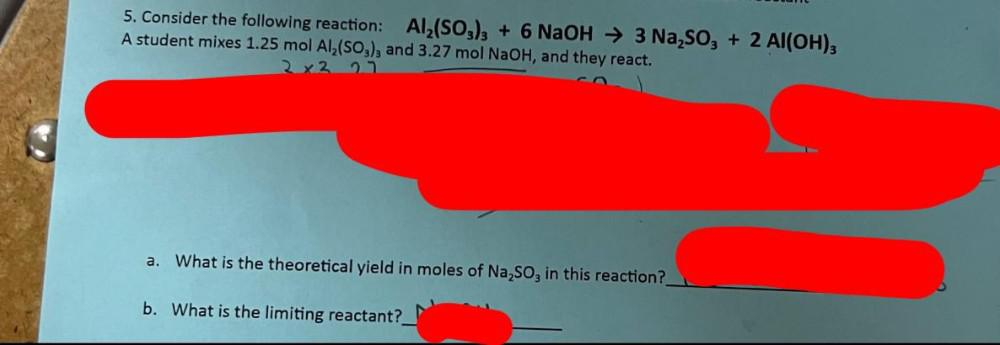Question:

# 5. Consider the following reaction: Al2(SO3)3 + 6 NaOH → 3 Na,, + 2 Al(OH)3 A student mixes 1.25 mol Al2(SO3), and 3.27 mol NaOH5. Consider the following reaction: Al2(SO3)3 + 6 NaOH → 3 Na,, + 2 Al(OH)3 A student mixes 1.25 mol Al2(SO3), and 3.27 mol NaOH, and they react. 2x2 23 a. What is the theoretical yield in moles of Na,, in this reaction? b. What is the limiting reactant?